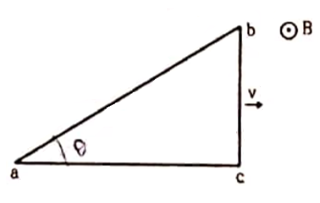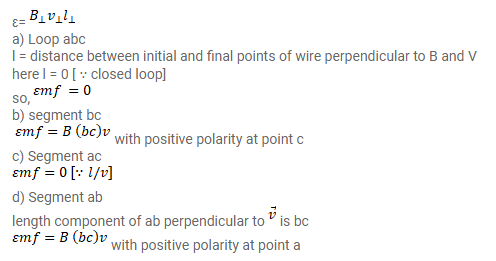# A right-angled triangle abc,

Question:

A right-angled triangle abc, made from a metallic wire, moves at a uniform speed $v$ as it is shown in figure. A uniform magnetic field B exists in the perpendicular direction. Find the emf induced

(a) in the loop abc,

(b) in the segment bc,

(c) in the segment ac and

(d) in the segment $a b$.Solution: